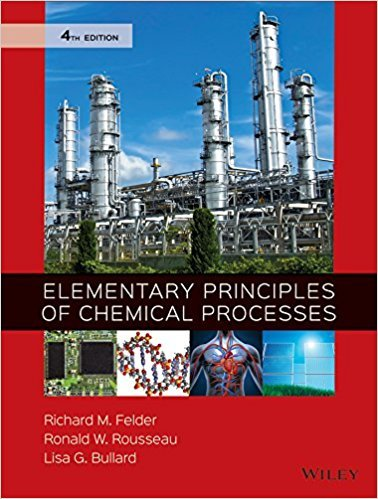×
×

# Solutions for Chapter A.2e: ITERATIVE SOLUTION OF NONLINEAR ALGEBRAIC EQUATIONS## Full solutions for Elementary Principles of Chemical Processes | 4th Edition

ISBN: 9780470616291Solutions for Chapter A.2e: ITERATIVE SOLUTION OF NONLINEAR ALGEBRAIC EQUATIONS

Solutions for Chapter A.2e
4 5 0 382 Reviews
20
0
##### ISBN: 9780470616291

This textbook survival guide was created for the textbook: Elementary Principles of Chemical Processes, edition: 4. Since 2 problems in chapter A.2e: ITERATIVE SOLUTION OF NONLINEAR ALGEBRAIC EQUATIONS have been answered, more than 38479 students have viewed full step-by-step solutions from this chapter. Elementary Principles of Chemical Processes was written by and is associated to the ISBN: 9780470616291. Chapter A.2e: ITERATIVE SOLUTION OF NONLINEAR ALGEBRAIC EQUATIONS includes 2 full step-by-step solutions. This expansive textbook survival guide covers the following chapters and their solutions.

Key Chemistry Terms and definitions covered in this textbook
• activation energy (Ea)

The minimum energy needed for reaction; the height of the energy barrier to formation of products. (Section 14.5)

• alkaloids

Naturally occurring amines isolated from plants.

• alkoxymercuration-demercuration

A two-step process that achieves Markovnikov addition of an alcohol (H and OR) across an alkene. The product of this process is an ether.

• amines.

Organic bases that have the functional group —NR2, where R may be H, an alkyl group, or an aromatic group. (24.4)

• Antiaromatic compound

A monocyclic compound that is planar or nearly so, has one 2p orbital on each atom of the ring, and has 4n p electrons in the cyclic arrangement of overlapping 2p orbitals, where n is an integer. Antiaromatic compounds are especially unstable

• asymmetric hydrogenation

The addition of H2 across only one face of a p bond.

• atomic orbital.

The wave function (?) of an electron in an atom. (7.5)

• chain reaction

A series of reactions in which one reaction initiates the next. (Section 21.7)

• Conjugate acid

The species formed when a base accepts a proton from an acid

• crystal lattice

An imaginary network of points on which the repeating motif of a solid may be imagined to be laid down so that the structure of the crystal is obtained. The motif may be a single atom or a group of atoms. Each lattice point represents an identical environment in the crystal. (Section 12.2)

• dimensional analysis

A method of problem solving in which units are carried through all calculations. Dimensional analysis ensures that the final answer of a calculation has the desired units. (Section 1.6)

• fibers

Strands of a polymer that are generated when the polymer is heated, forced through small holes, and then cooled.

• law of mass action

The rules by which the equilibrium constant is expressed in terms of the concentrations of reactants and products, in accordance with the balanced chemical equation for the reaction. (Section 15.2)

• Levorotatory

Refers to a substance that rotates the plane of polarized light to the left.

• nucleophilic aromatic substitution

A substitution reaction in which an aromatic ring is attacked by a nucleophile, which replaces a leaving group.

• nucleotides

The product formed when a nucleoside is coupled to a phosphate group

• rate constant

A constant of proportionality between the reaction rate and the concentrations of reactants that appear in the rate law. (Section 14.3)

• Syndiotactic polymer

A polymer with alternating R and S confi gurations at the chiral centers along its chain, as, for example, syndiotactic polypropylene

• trivalent

An element, such as nitrogen, that forms three bonds.

• Vinylic carbocation

A double-helix model for the secondary structure of a DNA molecule

×